# Understanding D.C. Motor CharacteristicsHome Intro How They Work CHARACTERISTICS Examples## Contents:

1. Preface
2. Background From Physics
3. 2.1 Torque
4. 2.2 Speed
5. 2.3 Power
6. Motor Characteristics
7. 3.1 Torque/Speed Curves
8. 3.2 Power Curves

*NOTE: Key terms are highlited in dark red.

## 1. Preface

This site deals with Direct Current permanent magnet motors operated at a constant voltage. Motor characteristics vary considerably from type to type, and their performance characteristics can be altered by the way electrical power is supplied. can be quite different than those covered here.

## 2. Background From Physics

### Section 2.1: TORQUE

Torque , as defined in © 1998 Merriam-Webster, Incorporated

Main Entry: torque
Function: noun
Etymology: Latin torquEre to twist
1 : a force that produces or tends to produce rotation or torsion (an automobile engine delivers torque to the drive shaft);
also : a measure of the effectiveness of such a force that consists of the product of the force and the perpendicular distance from the line of action of the force to the axis of rotation
2 : a turning or twisting force

Torque , as defined in University Physics, 8th ed. 1992, by Hugh D. Young:

The quantitative measure of the tendency of a force to cause or change rotational motion is called torque.

Torque (also called a moment) is the term we use when we talk about forces that act in a rotational manner. You apply a torque or moment when you turn a dial, flip a lightshwitch, drill a hole or tighten a screw or bolt.

As shown in the picture of a ratchet, a torque is created by a vertical force applied at the end of the handle. The force, F, applied to the ratchet as shown causes a tendency to rotate about point O. The force can be broken down into two components: a radial component, Frad, parallel to the ratchet handle that does not contribute to the torque, and a tangential component, Ftan, perpendicular to the handle that does contribute to the torque. The distance from point O to the point of action of F is described by the direction vector, r. The moment arm, l is the perpendicular distance between point O and the line of action of F.

If we were to shorten the moment arm by applying the force closer to the head of the ratchet,the magnitude of the torque would decrease, even if the force remained the same. Thus, if we change the effective length of the handle, we change the torque (see equation 1).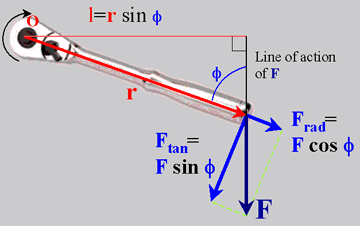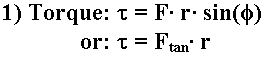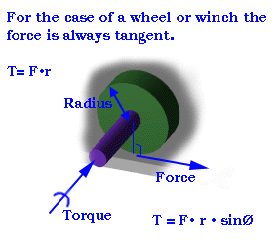UNITS of TORQUE
SI English
newton-meters {N·m} inch-pounds {in·lb}
foot-pounds {ft·lb}
inch-ounces {in·oz}
1 N·m = 0.738 ft·lb
1 N·m = 0.113 in·lb
1 N·m = 141.61 in·oz
1 in·lb = 0.113 N·m
1 ft·lb = 1.356 N·m
1 in·oz = 7.062E-03 N·m

Up to Contents

### Section 2.2: SPEED

Speed (Angular Velocity) , as defined in © 1998 Merriam-Webster, Incorporated

Main Entry: angular velocity
Function: noun
he rate of rotation around an axis usually expressed in radians or revolutions per second or per minute

Motors are devices that convert electrical energy into mechanical energy. The D.C. motors that we have been dealing with here convert electrical energy into rotational energy. That rotational energy is then used to lift things, propel things, turn things, etc... When we supply the specified voltage to a motor, it rotates the output shaft at some speed. This rotational speed or angular velocity,is typically measured in radians/second {rad/s}, revolutions/second {rps}, or revolutions/minute {rpm}.
• When performing calculations, be sure to use consistent units. In the English system, calculations should be done in degrees/second, and radians/sec for SI calculations.
 1 revolution = 360° 1 revolution = (2*p) radians 1 radian = (180/p)° 1° = (p/180) radians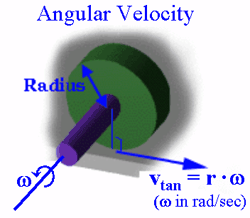From the angular velocity,, we can find the tangential velocity of a point anywhere on the rotating body through the equation tangential velocity, v = r*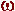, where r is the distance from the axis of rotation. This relation can be used to compute the steady state (constant speed - no acceleration) speed of a vehicle if the radius and angular velocity of a wheel is known, or the linear speed of a rope as it is wound up by a winch.

Up to Contents

### Section 2.3: POWER

Motive Power , as defined in © 1998 Merriam-Webster, Incorporated

Main Entry: 1pow�er
Pronunciation: 'pau(-&)r
Function: noun
Usage: often attributive
Etymology: Middle English, from Old French poeir, from poeir to be able, from (assumed) Vulgar Latin potEre, alteration of Latin posse
Date: 13th century
1 a : (1) : ability to act or produce an effect
6 a : a source or means of supplying energy; especially : ELECTRICITY
6 b : MOTIVE POWER c : the time rate at which work is done or energy emitted or transferred

Power in Rotational Motion , as described in University Physics, 8th ed., 1992 by Hugh D. Young):

When you pedal a bicycle, you apply forces to a rotating body and do work on it. Similar things happen in real-life situations, such as a rotating motor shaft driving a power tool or a car engine propelling the vehicle. We can express this work in terms of torque and an angular displacement...
What about the power associated with work done by a torque acting on a rotating body?

dW/dt is the rate of doing work, or power P. When a torque T (with respect to the axis of rotation) acts on a body that rotates with angular velocity W, its power (rate of doing work) is the product of the torque and angular velocity. This is the analog of the relation P = F·v for particle motion.

Power in rotational motion can be written as: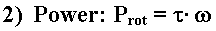SI English Watts {W} newton-meters per second {N·m/s} 1 W = 1 N·m/s 1 W = 0.738 ft·lb/s 1 W = 1.341E-03 hp foot-pounds per second {ft·lb/s} horsepower {hp} 1 ft·lb/s = 1.818E-03 hp 1 ft·lb/s = 1.356 W

Up to Contents

## 2. Motor Characteristics

### Section 3.1: TORQUE/SPEED CURVES

In order to effectively design with D.C. motors, it is necessary to understand their characteristic curves. For every motor, there is a specific Torque/Speed curve and Power curve.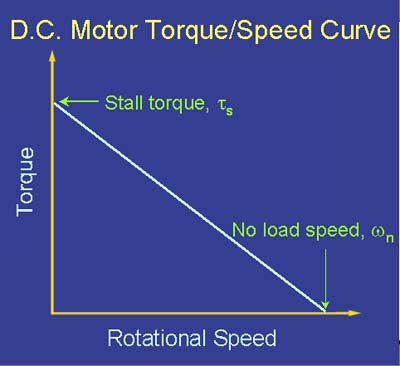The graph above shows a torque/speed curve of a typical D.C. motor. Note that torque is inversely proportioal to the speed of the output shaft. In other words, there is a tradeoff between how much torque a motor delivers, and how fast the output shaft spins. Motor characteristics are frequently given as two points on this graph:
• The stall torque,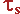, represents the point on the graph at which the torque is a maximum, but the shaft is not rotating.
• The no load speed,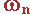, is the maximum output speed of the motor (when no torque is applied to the output shaft).
The curve is then approximated by connecting these two points with a line, whose equation can be written in terms of torque or angular velocity as equations 3) and 4):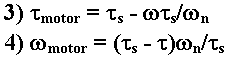The linear model of a D.C. motor torque/speed curve is a very good approximation. The torque/speed curves shown below are actual curves for the green maxon motor (pictured at right) used by students in 2.007. One is a plot of empirical data, and the other was plotted mechanically using a device developed at MIT. Note that the characteristic torque/speed curve for this motor is quite linear. This is generally true as long as the curve represents the direct output of the motor, or a simple gear reduced output. If the specifications are given as two points, it is safe to assume a linear curve.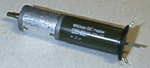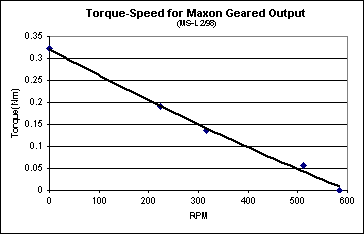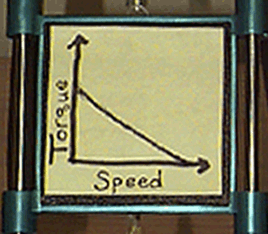Recall that earlier we defined power as the product of torque and angular velocity. This corresponds to the area of a rectangle under the torque/speed curve with one cornerat the origin and another corner at a point on the curve (see figures below). Due to the linear inverse relationship between torque and speed, the maximum power occurs at the point where= ½, and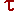= ½.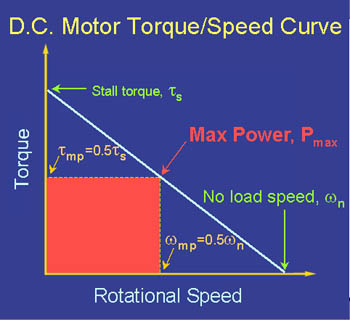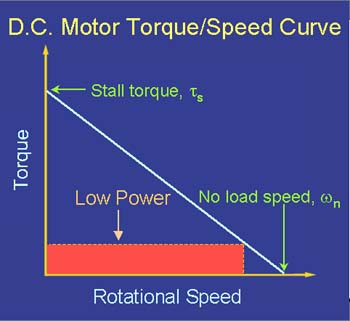Up to Contents

### Section 3.2: POWER/TORQUE and POWER/SPEED CURVES

By substituting equations 3. and 4. (torque and speed, section 2.1) into equation 2. (power, section 1.3), we see that the power curves for a D.C. motor with respect to both speed and torque are quadratics, as shown in equations 5. and 6.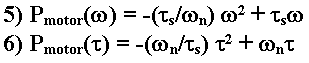From these equations, we again find that maximum output power occurs at= ½, and= ½repectively.Up to Contents

Home Intro How They Work CHARACTERISTICS Examples

Webmaster: Matt Page mpage1@mit.edu